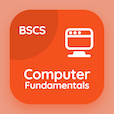Online CS Degree Courses

PHP MCQs

PHP MCQ - Topics

Learn Multidimensional Arrays Multiple Choice Questions (MCQ Quiz), Multidimensional Arrays quiz answers PDF to learn php course for php online classes. PHP Multidimensional Arrays Multiple Choice Questions and Answers (MCQs), Multidimensional Arrays quiz questions for online degrees. Multidimensional Arrays Book PDF: uses of arrays, iterations, multidimensional arrays test prep for information and communication technology.

"For finding nonempty elements in the array we use" MCQ PDF: multidimensional arrays App APK with is_array ( ) function, sizeof ( ) function, array_count ( ) function, and count ( ) function choices for online degrees. Study multidimensional arrays quiz questions for merit scholarship test and certificate programs for associates in computer science.

## MCQ on Multidimensional Arrays Quiz

MCQ: For finding nonempty elements in the array we use

is_array ( ) function
sizeof ( ) function
array_count ( ) function
count ( ) function

MCQ: The count ( ) function is identical to

is_array ( ) function
in_array ( ) function
sizeof ( )
isset ( ) function

MCQ: is_array ( ) function that takes a single argument of any type and returns a true value if

Argument is character type
Argument is number type
Argument is an array
none of them

MCQ: Which function take two arguments and returns true if element contained as a value of an array?

is_array ( ) function
in_array ( ) function
count ( ) function
isset ( ) function

MCQ: \$multi_array     = "I am working on PHP"; will create

One dimensional array
5 dimensional array
4 dimensional array
None of them

### More Quizzes on PHP Book